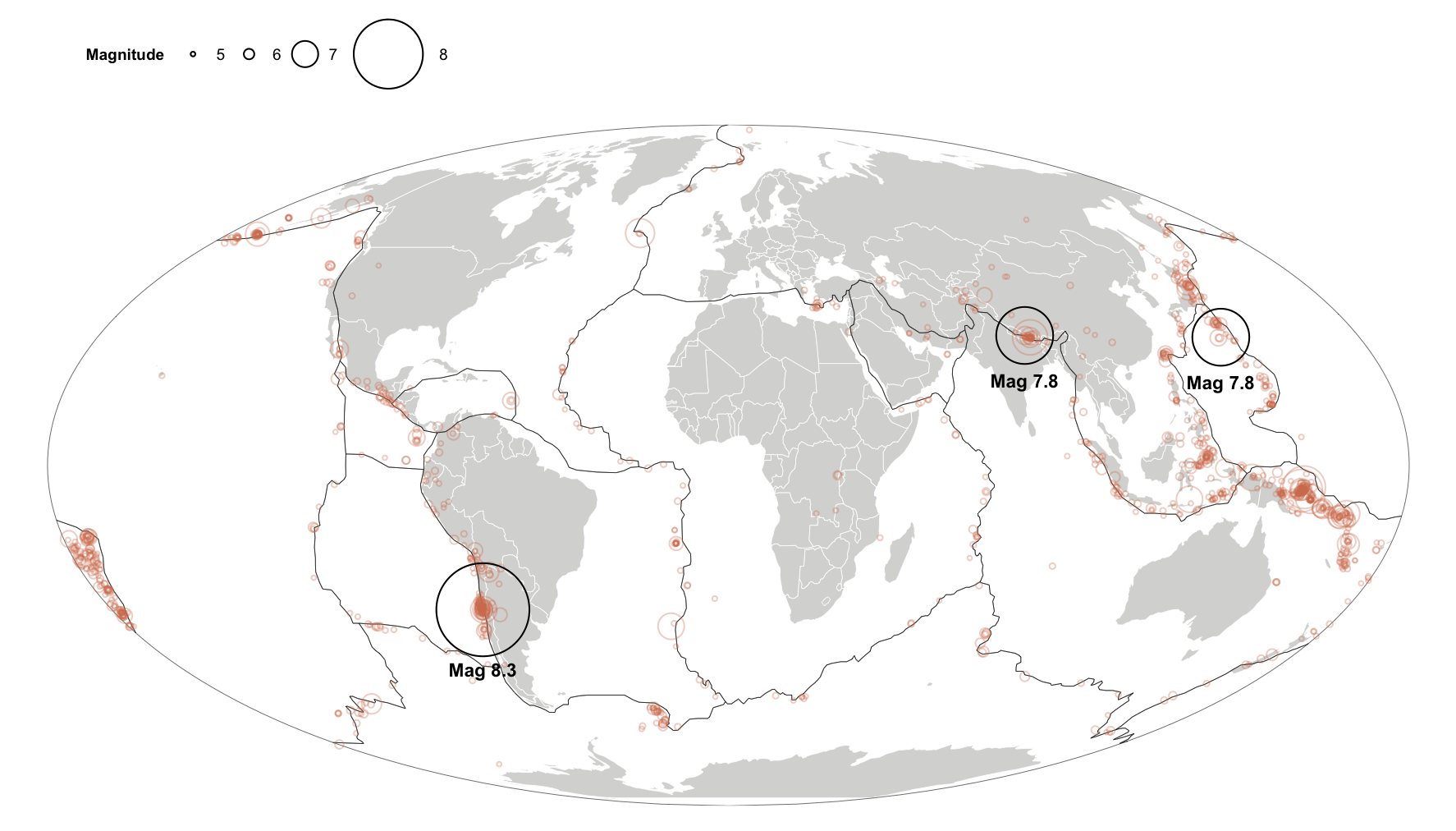Want to share your content on R-bloggers? click here if you have a blog, or here if you don't.

I saw this post over at NatGeo over the weekend and felt compelled to replicate this:with ggplot2.

Three shapefiles later and we have it close enough to toss into a post (and I really don’t believe the continent names are necessary).

```library(rgdal) library(ggplot2) library(ggthemes)   # grab these from http://rud.is/dl/quakefile.tgz   world <- readOGR("countries.geo.json", "OGRGeoJSON", stringsAsFactors=FALSE) plates <- readOGR("plates.json", "OGRGeoJSON", stringsAsFactors=FALSE) quakes <- readOGR("quakes.json", "OGRGeoJSON", stringsAsFactors=FALSE)   world_map <- fortify(world) plates_map <- fortify(plates) quakes_dat <- data.frame(quakes) quakes_dat\$trans <- quakes_dat\$mag %% 5   gg <- ggplot() gg <- gg + geom_map(data=world_map, map=world_map, aes(x=long, y=lat, map_id=id), color="white", size=0.15, fill="#d8d8d6") gg <- gg + geom_map(data=plates_map, map=plates_map, aes(x=long, y=lat, map_id=id), color="black", size=0.1, fill="#00000000", alpha=0) gg <- gg + geom_point(data=quakes_dat, aes(x=coords.x1, y=coords.x2, size=trans), shape=1, alpha=1/3, color="#d47e5d", fill="#00000000") gg <- gg + geom_point(data=subset(quakes_dat, mag>7.5), aes(x=coords.x1, y=coords.x2, size=trans), shape=1, alpha=1, color="black", fill="#00000000") gg <- gg + geom_text(data=subset(quakes_dat, mag>7.5), aes(x=coords.x1, y=coords.x2, label=sprintf("Mag %2.1f", mag)), color="black", size=3, vjust=c(3.9, 3.9, 5), fontface="bold") gg <- gg + scale_size(name="Magnitude", trans="exp", labels=c(5:8), range=c(1, 20)) gg <- gg + coord_map("mollweide") gg <- gg + theme_map() gg <- gg + theme(legend.position=c(0.05, 0.99)) gg <- gg + theme(legend.direction="horizontal") gg <- gg + theme(legend.key=element_rect(color="#00000000")) gg```I can only imagine how many mouse clicks that would be in a GIS program.